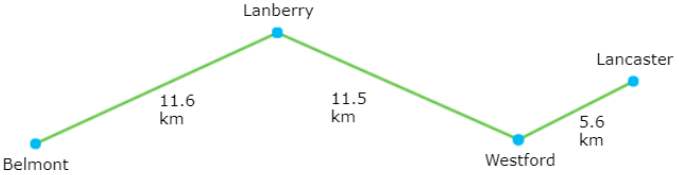Middle School Math, Warm-Up #2
Word Problems
In this section, for each question imagine you are writing equations for a word problem.
You are told there is an increase in volume by 1 L. Which number will you use in your formula?
1 point
You are told there is a 4km decrease in distance. Which number will you use in your formula?
1 point
You are told something happens 2m above sea level. Which number will you use in your formula?
1 point
You are told a student gets a penalty of 5 points in a game. Which number will you use in your formula?
1 point
Robert took \$20 out of his bank account. As a result the account now has \$3,459 left in it. How much money was in the account before the transfer?
1 point
Using the paths shown, how far is it from Belmont to Westford?
1 pointA factory makes ice cream. For the past 2 days the factory has made 4,716 cartons of ice cream each day. How many cartons of ice cream did the factory make in all?
1 point